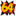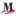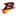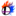A few useful how-tos for prospective dataminers. This is the basic stuff that is applicable to all games. Ask on the talk page if something seems wrong, missing, or needs a better explanation.

Computers can't count to 10; they can only count to 2. Therefore, all information in a computer system is stored in binary: every digit is either 0 or 1. For example, the number 361 is actually 0b101101001. (Note that adding "0b" to a number tells you it's in binary and not decimal.)

While it's important to recognize that everything is in binary, it's too long and unwieldy for most uses; note how 361 in binary is nine digits long. Therefore, we usually use hexadecimal, a number system that can count to 16 in one digit. This basically turns 4 binary digits into 1 hex digit, making things a lot shorter; 361 = 0x169 (note the "0x").

 Decimal Binary Hexadecimal 0 1 2 3 4 5 6 7 8 9 10 11 12 13 14 15 0000 0001 0010 0011 0100 0101 0110 0111 1000 1001 1010 1011 1100 1101 1110 1111 0 1 2 3 4 5 6 7 8 9 A B C D E F

In the Smash Bros. games, numbers are usually eight hex digits long, or four bytes. This makes 0xFFFFFFFF = 4,294,967,295 the largest possible integer.

## Negative numbers

Fundamentally, computers can only count up from zero. So to represent negative numbers, we need a trick. The basic idea of the trick follows this thought process:

• "We need negative numbers."
• "4,294,967,295 is a really big number. We don't really need numbers that big."
• "We can get away with the biggest number being half that, or 2,147,483,647."
• "Let's cut the available integers in half. The top half will be negative."

As a result, instead of the possible range being [0 - 4,294,967,295], it's [-2,147,483,648 - +2,147,483,647]. (It's one number bigger on the negative half because 0 ends up being part of the positive half.)

So how do you tell if a number is supposed to be negative? Easy: its first bit is 1. Or to put it another way, its first hex digit is between 8 and F. Just like most positive numbers start with a lot of 0s and then the value itself, most negative numbers will start with a lot of Fs; 10 = 0x0000000A and -10 = 0xFFFFFFF6.

Once you recognize that a hex number is negative, invert all its bits and add 1 to get the negated value. You can also get its value by putting this into Google:

`0x100000000 - 0x######## in decimal`

(Note how the number used has 8 zeros, which is because the number being checked has 8 digits.)

## Numbers with decimal points (floats)

Computers can only count on their fingers. We need another trick to work with numbers that have decimal points (like 2.5). It's a pretty complicated trick, but the basic idea is that we split up the number into multiple parts and do some funky math on them, getting a range of numbers that's decent enough for most purposes. Note that Smash Bros. exclusively uses single-precision floats.

It's pretty easy to recognize whether a hex number is a float, at least under the assumption that you're working with reasonable numbers. They start with the hex digits 3 or 4, and in most cases end with a repeating digit. For example, 0x3F800000 = 1.0; 0x40000000 = 2.0; 0x42fA0000 = 125.0; 0x3E99999A = 0.3 (note that you may have to recognize this is supposed to be rounded to 0.3, as computers can only get a number "perfect" if it can be reached by dividing by 2). Negatives start with B or C and are simpler to convert than integers: just swap 4 with C and 3 with B. 0x41480000 = 12.5; 0xC1480000 = -12.5.

While you may learn to identify some specific values, the only reasonable way to convert back and forth from hex to decimal is to find an IEEE-754 applet on the internet or something. Toomai uses this one.

Note that if a number is 0, you can't tell whether it's an integer or a float because they look the same.

## Game-specific info